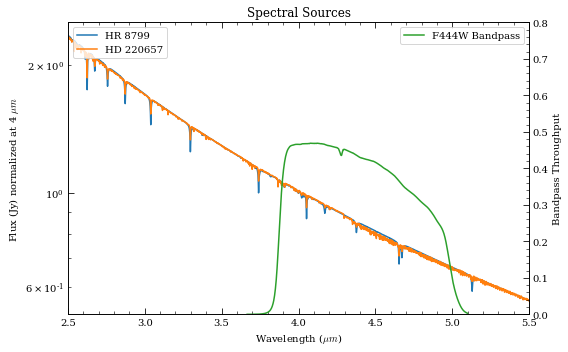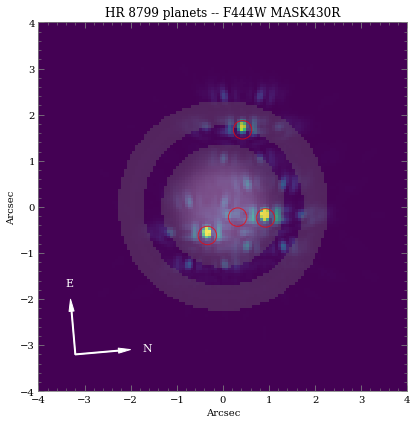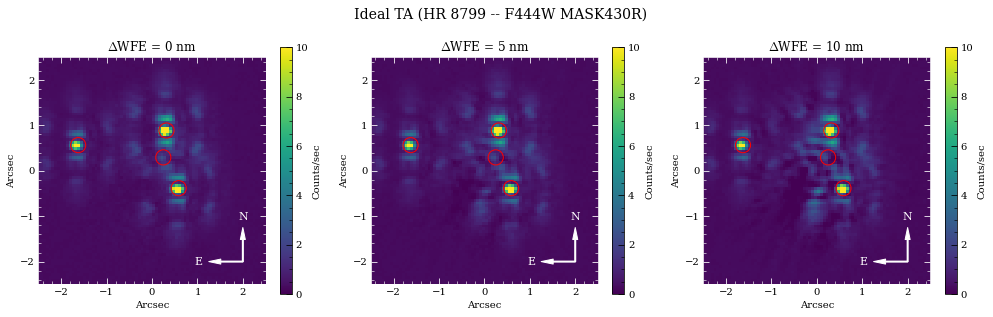# Coronagraph Basics¶

This set of exercises guides the user through a step-by-step process of simulating NIRCam coronagraphic observations of the HR 8799 exoplanetary system. The goal is to familiarize the user with basic pynrc classes and functions relevant to coronagraphy.

:

# If running Python 2.x, makes print and division act like Python 3
from __future__ import print_function, division

# Import the usual libraries
import numpy as np
import matplotlib
import matplotlib.pyplot as plt

# Enable inline plotting at lower left
%matplotlib inline

from IPython.display import display, Latex, clear_output


We will start by first importing pynrc along with the obs_hci (High Contrast Imaging) class, which lives in the pynrc.obs_nircam module.

:

import pynrc
from pynrc import nrc_utils          # Variety of useful functions and classes
from pynrc.obs_nircam import obs_hci # High-contrast imaging observation class

# Disable informational messages and only include warnings and higher
pynrc.setup_logging(level='WARN')

pyNRC log messages of level WARN and above will be shown.
pyNRC log outputs will be directed to the screen.


## Source Definitions¶

The obs_hci class first requires two arguments describing the spectra of the science and reference sources (sp_sci and sp_ref, respectively. Each argument should be a Pysynphot spectrum already normalized to some known flux. pynrc includes built-in functions for generating spectra. The user may use either of these or should feel free to supply their own as long as it meets the requirements.

1. The pynrc.stellar_spectrum function provides the simplest way to define a new spectrum:

bp_k = pynrc.bp_2mass('k') # Define bandpass to normalize spectrum
sp_sci = pynrc.stellar_spectrum('F0V', 5.24, 'vegamag', bp_k)


You can also be more specific about the stellar properties with Teff, metallicity, and log_g keywords.

sp_sci = pynrc.stellar_spectrum('F0V', 5.24, 'vegamag', bp_k,
Teff=7430, metallicity=-0.47, log_g=4.35)

2. Alternatively, the pynrc.source_spectrum class ingests spectral information of a given target and generates a model fit to the known photometric SED. Two model routines can be fit. The first is a very simple scale factor that is applied to the input spectrum, while the second takes the input spectrum and adds an IR excess modeled as a modified blackbody function. The user can find the relevant photometric data at http://vizier.u-strasbg.fr/vizier/sed/ and click download data as a VOTable.

:

# Define 2MASS Ks bandpass and source information
bp_k = pynrc.bp_2mass('k')

# Science         source,  dist, age, sptype, Teff, [Fe/H], log_g, mag, band
args_sources = [('HR 8799', 39.0, 30,  'F0V', 7430, -0.47, 4.35, 5.24, bp_k)]

# References     source,     sptype, Teff, [Fe/H], log_g, mag, band
ref_sources = [('HD 220657', 'F8III', 5888, -0.01, 3.22, 3.04, bp_k)]

:

name_sci, dist_sci, age, spt_sci, Teff_sci, feh_sci, logg_sci, mag_sci, bp_sci = args_sources
name_ref, spt_ref, Teff_ref, feh_ref, logg_ref, mag_ref, bp_ref = ref_sources

# For the purposes of simplicity, we will use pynrc.stellar_spectrum()
sp_sci = pynrc.stellar_spectrum(spt_sci, mag_sci, 'vegamag', bp_sci,
Teff=Teff_sci, metallicity=feh_sci, log_g=logg_sci)
sp_sci.name = name_sci

# And the refernece source
sp_ref = pynrc.stellar_spectrum(spt_ref, mag_ref, 'vegamag', bp_ref,
Teff=Teff_ref, metallicity=feh_ref, log_g=logg_ref)
sp_ref.name = name_ref

:

# Plot the two spectra
fig, ax = plt.subplots(1,1, figsize=(8,5))

xr = [2.5,5.5]

for sp in [sp_sci, sp_ref]:
w = sp.wave / 1e4
ind = (w>=xr) & (w<=xr)
sp.convert('Jy')
f = sp.flux / np.interp(4.0, w, sp.flux)
ax.semilogy(w[ind], f[ind], lw=1.5, label=sp.name)
ax.set_ylabel('Flux (Jy) normalized at 4 $\mu m$')
sp.convert('flam')

ax.set_xlim(xr)
ax.set_xlabel(r'Wavelength ($\mu m$)')
ax.set_title('Spectral Sources')

# Overplot Filter Bandpass
ax2 = ax.twinx()
ax2.plot(bp.wave/1e4, bp.throughput, color='C2', label=bp.name+' Bandpass')
ax2.set_ylim([0,0.8])
ax2.set_xlim(xr)
ax2.set_ylabel('Bandpass Throughput')

ax.legend(loc='upper left')
ax2.legend(loc='upper right')

fig.tight_layout()## Initialize Observation¶

Now we will initialize the high-contrast imaging class pynrc.obs_hci using the spectral objects and various other settings. The obs_hci object is a subclass of the more generalized NIRCam class. It implements new settings and functions specific to high-contrast imaging observations for corongraphy and direct imaging.

For this tutorial, we want to observe these targets using the MASK430R coronagraph in the F444W filter. All circular coronagraphic masks such as the 430R (R=round) should be paired with the CIRCLYOT pupil element, whereas wedge/bar masks are paired with WEDGELYOT pupil. Observations in the LW channel are most commonly observed in WINDOW mode with a 320x320 detector subarray size. Full detector sizes are also available.

The PSF simulation size (fov_pix keyword) should also be of similar size as the subarray window (recommend avoiding anything above fov_pix=1024 due to computation time and memory usage). Use odd numbers to center the PSF in the middle of the pixel. If fov_pix is specified as even, then PSFs get centered at the corners. This distinction really only matter for unocculted observations, (ie., where the PSF flux is concentrated in a tight central core).

We also need to specify a WFE drift value (wfe_ref_drift parameter), which defines the anticipated drift in nm between the science and reference sources. For the moment, let’s intialize with a value of 0nm. This prevents an initially long process by which pynrc calculates changes made to the PSF over a wide range of drift values.

Extended disk models can also be specified upon initialization using the disk_hdu keyword.

:

filt, mask, pupil = ('F444W',  'MASK430R', 'CIRCLYOT')
wind_mode, subsize = ('WINDOW', 320)
fov_pix, oversample = (320, 2)

wfe_ref_drift = 0
wfe_ref_drift=wfe_ref_drift, fov_pix=fov_pix, oversample=oversample,
wind_mode=wind_mode, xpix=subsize, ypix=subsize, verbose=True)

Generating background PSF coefficients...
Generating oversampled PSFs...
Updating NIRCam reference coefficients...
Creating NIRCam reference class...
Finished.


All information for the reference observation is stored in the attribute obs.nrc_ref, which is simply it’s own isolated NIRCam (nrc_hci) class. After initialization, any updates made to the primary obs instrument configuration (e.g., filters, detector size, etc.) must also be made inside the obs.nrc_ref class as well. That is to say, it does not automatically propogate. In many ways, it’s best to think of these as two separate classes,

obs_sci = obs
obs_ref = obs.nrc_ref


with some linked references between the two.

Now that we’ve succesffully initialized the obs_hci observations, let’s specify the wfe_ref_drift. If this is your first time, then the nrc_utils.wfed_coeff function is called to determine a relationship between PSFs in the presense of WFE drift. This relationship is saved to disk in the PYNRC_DATA directory as a set of polynomial coefficients. Future calculations utilize these coefficients to quickly generate a new PSF for any arbitary drift value.

:

# WFE drift amount between rolls
# This only gets called during gen_roll_image()
# and temporarily updates obs.wfe_drift to create
# a new PSF.
obs.wfe_roll_drift = 2

# Drift amount between Roll 1 and reference
# This is simply a link to obs.nrc_ref.wfe_drift
obs.wfe_ref_drift = 10


## Exposure Settings¶

Optimization of exposure settings are demonstrated in another tutorial, so we will not repeat that process here. We can assume the optimization process was performed elsewhere to choose the DEEP8 pattern with 16 groups and 5 total integrations. These settings apply to each roll position of the science observation as well as the for the reference observation.

:

# Update both the science and reference observations

New Ramp Settings:
nf        :        8
nd2       :       12
ngroup    :       16
nint      :        5
New Detector Settings
wind_mode :   WINDOW
xpix      :      320
ypix      :      320
x0        :      914
y0        :     1513
New Ramp Times
t_group   :   21.381
t_frame   :    1.069
t_int     :  329.264
t_int_tot :  330.353
t_exp     : 1646.322
t_acq     : 1651.766


There are four known giant planets orbiting HR 8799 at various locations. Ideally, we would like to position them at their predicted locations on the anticipated observation date. For this case, we choose a plausible observation date of November 1, 2019. To convert between $$(x,y)$$ and $$(r,\theta)$$, use the nrc_utils.xy_to_rtheta and nrc_utils.rtheta_to_xy functions.

When adding the planets, it doesn’t matter too much which exoplanet model spectrum we decide to use since the spectra are still fairly unconstrained at these wavelengths. We do know roughly the planets’ luminosities, so we can simply choose some reasonable model and renormalize it to the appropriate filter brightness. Currently, the only exoplanet spectral models available to pynrc are those from Spiegel & Burrows (2012).

:

# Projected locations for date 11/01/2019
# These are prelimary positions, but within constrained orbital parameters
loc_list = [(-1.57, 0.64), (0.42, 0.87), (0.5, -0.45), (0.35,  0.20)]

# Estimated magnitudes within F444W filter
pmags = [16.0, 15.0, 14.6, 14.7]

:

# Add planet information to observation class.
# These are stored in obs.planets.
# Can be cleared using obs.kill_planets().
obs.kill_planets()
for i, loc in enumerate(loc_list):
renorm_args=(pmags[i], 'vegamag', obs.bandpass))

:

# Generate and plot a noiseless slope image to make sure things look right
PA1 = 85
im_planets = obs.gen_planets_image(PA_offset=PA1)

:

from matplotlib.patches import Circle

fig, ax = plt.subplots(figsize=(6,6))

xasec = obs.det_info['xpix'] * obs.pix_scale
yasec = obs.det_info['ypix'] * obs.pix_scale
extent = [-xasec/2, xasec/2, -yasec/2, yasec/2]
xylim = 4

vmin = 0
vmax = 0.5*im_planets.max()
ax.imshow(im_planets, extent=extent, vmin=vmin, vmax=vmax)

detid = obs.Detectors.detid
# Do some masked transparency overlays

xc_off = obs.bar_offset
for loc in loc_list:
xc, yc = loc
xc, yc = nrc_utils.xy_rot(xc, yc, PA1)
xc += xc_off
circle = Circle((xc,yc), radius=xylim/15., alpha=0.7, lw=1, edgecolor='red', facecolor='none')

xlim = ylim = np.array([-1,1])*xylim
xlim = xlim + xc_off
ax.set_xlim(xlim)
ax.set_ylim(ylim)

ax.set_xlabel('Arcsec')
ax.set_ylabel('Arcsec')

ax.set_title('{} planets -- {} {}'.format(sp_sci.name, obs.filter, obs.mask))

color = 'grey'
ax.tick_params(axis='both', color=color, which='both')
for k in ax.spines.keys():
ax.spines[k].set_color(color)

position=(0.25,0.9), label1='E', label2='N')

fig.tight_layout()As we can see, even with “perfect PSF subtraction” and no noise, it’s difficult to make out planet e. This is primarily due to its location relative to the occulting mask reducing throughput along with confusion of bright diffraction spots from nearby sources.

## Estimated Performance¶

Now we are ready to determine contrast performance and sensitivites as a function of distance from the star.

### 1. Roll-Subtracted Images¶

First, we will create a quick simulated roll-subtracted image using the in gen_roll_image method. For the selected observation date of 11/1/2019, APT shows a PA range of 84$$^{\circ}$$ to 96$$^{\circ}$$. So, we’ll assume Roll 1 has PA1=85, while Roll 2 has PA2=95. In this case, “roll subtraction” simply creates two science images observed at different parallactic angles, then subtracts the same reference observation from each. The two results are then de-rotated to a common PA=0 and averaged.

There is also the option to create ADI images, where the other roll position becomes the reference star by setting no_ref=True.

:

# Cycle through a few WFE drift values
wfe_list = [0,5,10]

# PA values for each roll
PA1, PA2 = (85, 95)

# A dictionary of HDULists
hdul_dict = {}
for i, wfe_drift in enumerate(wfe_list):
print(wfe_drift)
# Upate WFE reference drift value
obs.wfe_ref_drift = wfe_drift

# Set the final output image to be oversampled
hdulist = obs.gen_roll_image(PA1=PA1, PA2=PA2)
hdul_dict[wfe_drift] = hdulist


0
5
10

:

from pynrc.obs_nircam import plot_hdulist
from matplotlib.patches import Circle

fig, axes = plt.subplots(1,3, figsize=(14,4.3))
xylim = 2.5
xlim = ylim = np.array([-1,1])*xylim

for j, wfe_drift in enumerate(wfe_list):
ax = axes[j]
hdul = hdul_dict[wfe_drift]

plot_hdulist(hdul, xr=xlim, yr=ylim, ax=ax, vmin=0, vmax=8)

# Location of planet
for loc in loc_list:
circle = Circle(loc, radius=xylim/15., lw=1, edgecolor='red', facecolor='none')

ax.set_title('$\Delta$WFE = {:.0f} nm'.format(wfe_drift))

nrc_utils.plotAxes(ax, width=1, headwidth=5, alength=0.15, position=(0.9,0.7), label1='E', label2='N')

fig.suptitle('{} -- {} {}'.format(name_sci, obs.filter, obs.mask), fontsize=14)
fig.tight_layout()Note: At first glance, it appears as if the innermost Planet e is getting brighter with increased WFE drift, which would be understandably confusing. However, upon further investigation, there just happens to be a bright residual speckle that lines up well with Planet e when observed at this specific parallactic angle. This was verified by adjusting the observed PA as well as removing the planets from the simulations.

### 2. Contrast Curves¶

Next, we will cycle through a few WFE drift values to get an idea of potential predicted sensitivity curves. The calc_contrast method returns a tuple of three arrays: 1. The radius in arcsec. 2. The n-sigma contrast. 3. The n-sigma magnitude sensitivity limit (vega mag).

:

# Cycle through varying levels of WFE drift and calculate contrasts
wfe_list = [0,5,10]
nsig = 5

# PA values for each roll
PA1, PA2 = (85, 95)
roll_angle = np.abs(PA2 - PA1)

curves = []
for i, wfe_drift in enumerate(wfe_list):
print(wfe_drift)
# Generate series of observations for each filter
obs.wfe_ref_drift = wfe_drift

# Generate contrast curves
result = obs.calc_contrast(roll_angle=roll_angle, nsig=nsig)
curves.append(result)


0
5
10

:

from pynrc.obs_nircam import plot_contrasts, plot_planet_patches, plot_contrasts_mjup
import matplotlib.patches as mpatches

# fig, ax = plt.subplots(figsize=(8,5))
fig, axes = plt.subplots(1,2, figsize=(14,4.5))
xr=[0,5]
yr=[24,8]

# 1a. Plot contrast curves and set x/y limits
ax = axes
ax, ax2, ax3 = plot_contrasts(curves, nsig, wfe_list, obs=obs,
xr=xr, yr=yr, ax=ax, return_axes=True)
# 1b. Plot the locations of exoplanet companions
label = 'Companions ({})'.format(filt)
planet_dist = [np.sqrt(x**2+y**2) for x,y in loc_list]
ax.plot(planet_dist, pmags, marker='o', ls='None', label=label, color='k', zorder=10)

# 1c. Plot Spiegel & Burrows (2012) exoplanet fluxes (Hot Start)
plot_planet_patches(ax, obs, age=age, entropy=13, av_vals=None)
ax.legend(ncol=2)

# 2. Plot in terms of MJup using COND models
ax = axes
plot_contrasts_mjup(curves, nsig, wfe_list, obs=obs, age=age,
ax=ax, twin_ax=True, xr=xr, yr=None)
ax.set_yscale('log')
ax.set_ylim([0.08,100])
ax.legend(loc='upper right', title='COND ({:.0f} Myr)'.format(age))

fig.suptitle('{} ({} + {})'.format(name_sci, obs.filter, obs.mask), fontsize=16)

fig.tight_layout()The innermost Planet e is right on the edge of the detection threshold as suggested by the simulated images.

### 3. Saturation Levels¶

Create an image showing level of saturation for each pixel. For NIRCam, saturation is important to track for purposes of accurate slope fits and persistence correction. In this case, we will plot the saturation levels both at NGROUP=2 and NGROUP=obs.det_info['ngroup']. Saturation is defined at 80% well level, but can be modified using the well_fill keyword.

We want to perform this analysis for both science and reference targets.

:

# Saturation limits
ng_max = obs.det_info['ngroup']
sp_flat = pynrc.stellar_spectrum('flat')

print('NGROUP=2')
_ = obs.sat_limits(sp=sp_flat,ngroup=2,verbose=True)

print('')
print('NGROUP={}'.format(ng_max))
_ = obs.sat_limits(sp=sp_flat,ngroup=ng_max,verbose=True)

mag_sci = obs.star_flux('vegamag')
mag_ref = obs.star_flux('vegamag', sp=obs.sp_ref)
print('')
print('{} flux at {}: {:0.2f} mags'.format(obs.sp_sci.name, obs.filter, mag_sci))
print('{} flux at {}: {:0.2f} mags'.format(obs.sp_ref.name, obs.filter, mag_ref))

NGROUP=2
F444W Saturation Limit assuming Flat spectrum in photlam source: 2.35 vegamag

NGROUP=16
F444W Saturation Limit assuming Flat spectrum in photlam source: 4.95 vegamag

HR 8799 flux at F444W: 5.24 mags
HD 220657 flux at F444W: 3.03 mags


In this case, we don’t expect HR 8799 to saturated. However, the reference source should have some saturated pixels before the end of an integration.

:

# Well level of each pixel for science source
sci_levels1 = obs.saturation_levels(ngroup=2)
sci_levels2 = obs.saturation_levels(ngroup=ng_max)

# Which pixels are saturated?

:

# Well level of each pixel for reference source
ref_levels1 = obs.saturation_levels(ngroup=2, do_ref=True)
ref_levels2 = obs.saturation_levels(ngroup=ng_max, do_ref=True)

# Which pixels are saturated?

:

# How many saturated pixels?

print(obs.sp_sci.name)
print('{} saturated pixel at NGROUP=2'.format(nsat1_sci))
print('{} saturated pixel at NGROUP={}'.format(nsat2_sci,ng_max))

# How many saturated pixels?

print('')
print(obs.sp_ref.name)
print('{} saturated pixel at NGROUP=2'.format(nsat1_ref))
print('{} saturated pixel at NGROUP={}'.format(nsat2_ref,ng_max))

HR 8799
0 saturated pixel at NGROUP=2
0 saturated pixel at NGROUP=16

HD 220657
0 saturated pixel at NGROUP=2
719 saturated pixel at NGROUP=16

:

# Saturation Mask for science target

nsat1, nsat2 = (nsat1_sci, nsat2_sci)
sp = obs.sp_sci
nrc = obs

# Only display saturation masks if there are saturated pixels
if nsat2 > 0:
fig, axes = plt.subplots(1,2, figsize=(10,5))

xasec = nrc.det_info['xpix'] * nrc.pix_scale
yasec = nrc.det_info['ypix'] * nrc.pix_scale
extent = [-xasec/2, xasec/2, -yasec/2, yasec/2]

axes.set_title('{} Saturation (NGROUP=2)'.format(sp.name))
axes.set_title('{} Saturation (NGROUP={})'.format(sp.name,ng_max))

for ax in axes:
ax.set_xlabel('Arcsec')
ax.set_ylabel('Arcsec')

ax.tick_params(axis='both', color='white', which='both')
for k in ax.spines.keys():
ax.spines[k].set_color('white')

fig.tight_layout()
else:
print('No saturation detected.')

No saturation detected.

:

# Saturation Mask for reference

nsat1, nsat2 = (nsat1_ref, nsat2_ref)
sp = obs.sp_ref
nrc = obs.nrc_ref

# Only display saturation masks if there are saturated pixels
if nsat2 > 0:
fig, axes = plt.subplots(1,2, figsize=(10,5))

xasec = nrc.det_info['xpix'] * nrc.pix_scale
yasec = nrc.det_info['ypix'] * nrc.pix_scale
extent = [-xasec/2, xasec/2, -yasec/2, yasec/2]

axes.set_title('{} Saturation (NGROUP=2)'.format(sp.name))
axes.set_title('{} Saturation (NGROUP={})'.format(sp.name,ng_max))

for ax in axes:
ax.set_xlabel('Arcsec')
ax.set_ylabel('Arcsec')

ax.tick_params(axis='both', color='white', which='both')
for k in ax.spines.keys():
ax.spines[k].set_color('white')

fig.tight_layout()
else:
print('No saturation detected.')[ ]: